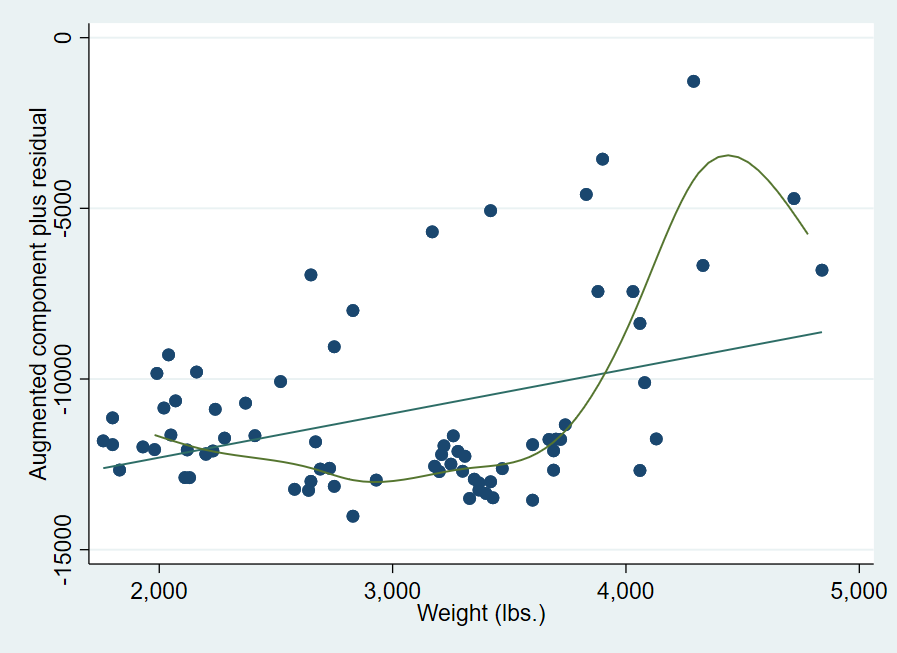Search

# Stata中的模型诊断图之偏残差图以及非线性回归简述

Updated: Apr 1, 2021

```cprplot independent_variable
acprplot independent_variable

```

```cprplot independent_variable, lowess
acprplot independent_variable, lowess```

```cprplot independent_variable, mspline
acprplot independent_variable, mspline```

```sysuse auto
regress price mpg weight```

```cprplot mpg, lowess
acprplot mpg, lowess
cprplot mpg, mspline
acprplot mpg, mspline``````cprplot weight, lowess
acprplot weight, lowess
cprplot weight, mspline
acprplot weight, mspline```